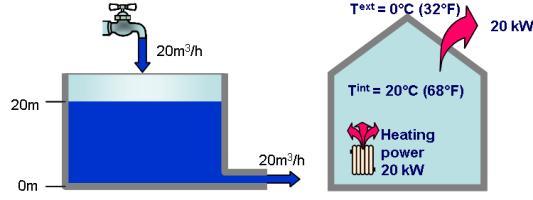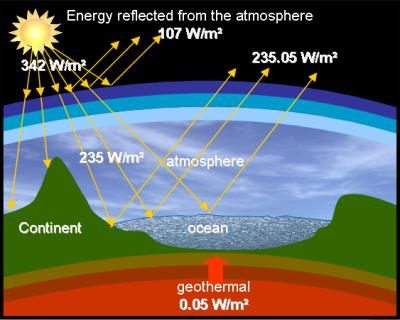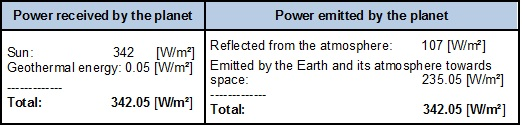Chargement...

# 2) The thermal equilibrium of the planet 2) The thermal equilibrium of the planet

2) The thermal equilibrium of the planet

Let’s begin by developing our “common sense” of thermal science.

Let’s remember the general principle of heating .

We can compare a heated building to a reservoir:• The level of water in the reservoir remains constant if there is as much water coming in as there is coming out
• The temperature level of the house remains constant if there is as much heat coming in as there is coming out (this can also be stated as “ the temperature level of the house remains constant if there is as much heat coming out as there is coming in”)

As such, the temperature of the Earth remains constant as long as it emits as much heat as it receives from outside (from the sun) or from inside (from the center of the Earth or from human activity).

Prior to the current Global Warming period, the situation of the planet could be represented by the following scheme: